# LOG#066. SM(VIII): tests.

##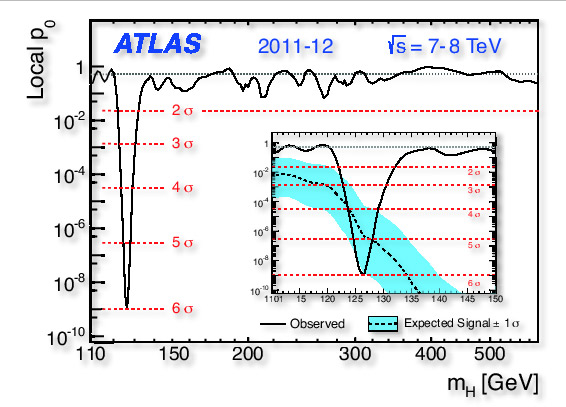## The weak scale and the weak angle

The Fermi constant is defined through a beautiful and simple mathematical formula: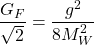This formula for the Fermi constant was very important in the long path towards the EW unification since knowing the Fermi constant allows to guess or estimate the value of the W-mass! Moreover, this constant is also determined independently by experimental muon lifetime, and it yields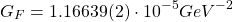The weak scale or equivalently the v.e.v. of the physical Higgs field is given by: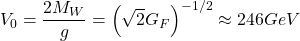Similarly, we also have a relation between the electric charge and the weak coupling constant via the Weinberg angle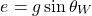To the lowest order (tree level in QFT calculations), the W-boson mass and the Z-boson mass are related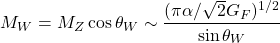where the electromagnetic fine structure constant is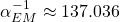Experimentally, the Weinberg angle (more precisely its sine or cosine) is determined experimentally from the neutral current scattering experiments, and they provide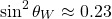## The Higgs mass: limits and 2012 discovery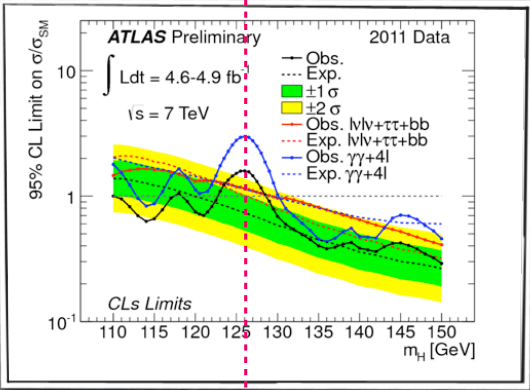Before 2012, theoretical physicists had only theoretical hints about the possible Higgs mass values. The Higgs mass was not predicted by the SM, BUT some general principles based on QM, QFT and preliminary results in collider physics provided the next bounds:

1) We knew experimental lower limits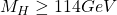and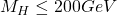from LEP (CERN) , CDF (FermiLAB, TeVatron), and the precision measurements of the SM observables, that strongy pointed out towards a “low Higgs mass”.

2) There were strong and theoretical bounds in the “very low” Higgs mass range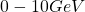. Despite the fact they depended on the top quark mass, they strongly suggested that the Higgs mass could not be “very low” in energy. It would have been detected!

3) Some general arguments for strongly coupled Higgs particles, related to the Higgs-self-coupling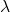strongly suggested that the Higgs particle could not be heavier than.

4) Unitarity in the s-channel scattering for the Higgs bosons suggested that the Higgs boson could not be heavier than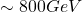.

There were some other highly technical bounds on the Higgs mass, but I am not going to discuss it here. There are plenty of books and lectures covering that topic. I am only interested in the master lines of the Higgs hunters…Until this wonderful year. 2012 will be remembered, and this summer particularly, due to the discovery of the Higgs particle ( or something that looks like a Higgs boson, but we have to wait for that claim) by ATLAS and CMS, the two main collaborations in the LHC(Large Hadron Collider, for short). The LHC, a 27 Km hadron-hadron (proton-proton) collider claimed this summer they have found a new boson particle or “resonance” around the mass:It is consistent with theoretical and previous experiments/tests but…What value do you prefer? A cubic Higgs mass value? Maybe a magical number likeGeV?Or maybe you prefer…A prime number like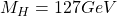. Well, I prefer prime numbers (like the W and Z bosons, closely to 79 and 91 respectively in GeV units), so I like the Higgs mass to be! Indeed, 127 is close to 128, and 128 is also interesting since it is almost the inverse of the electromagnetic fine structure constant at the Z-pole mass! If we are tempted to related couplings to masses as they seem to be from mixing and ratios, this is a deep result or perhaps another extraordinary coincidence!

Moreover, in the end of the 2012 we have obtained strong suggestions that the 127 GeV particle has spin zero and it is very likely to be parity even! Spin zero is what we would expect from a SM Higgs and it would rule out weird spin 2 variants like some extra-dimensional impostors or Kaluza-Klein resonances and similar “cousins”. Being parity even, it also rules out some weird alternative beyond the Standard Model. So, it seems that whatever the New Physics is really very well hidden from our common eyes and vision. At least, till now. In summary, neglecting some disturbances in the ATLAS and CMS experiments, probably some systematic error, every data collected until 2012 is consistent with a SM Higgs. Are you worried as a SUSY/string believer? Perhaps you should not but data can not be denied! 😉 SUSY can hardly be the answer to the EW-Planck hierarchy problem if SUSY is broken. If it(SUSY) is broken at high energy it can not be the explanation of why the Higgs mass is light since it should receive a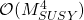correction that can not be easily explained without a fine-tuned SUSY. If it is not SUY, then what protects the Higgs mass to receive quantum corrections to its mass? Likely, it is one of the most appealing questions that the current SM leaves unsolved!

## Quark masses

From the 2012 PDG booklet we obtain: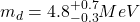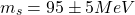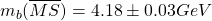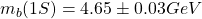Moreover, direct measurements provideand minimal substractionfrom cross-section measurements give.

We compare these values with the known fundamental masses for leptons: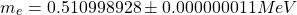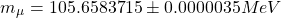Current values for neutrino masses are now known beyond experimental and theoretical bounds.

## Further experimental tests

The charged current interactions have ben succesfully tested both, experimentally and theoretically. Moreover, CC interactions have been tested in weak decays:

1st. Decays involving kaons, hyperons, heavy quarks, muons and tau particles.

2nd. Precision measurments of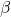decays, and/or decays from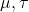particles are a sensitive probe of extended gauge groups involving right-handed currents and other types of new physics associated with new particles that we have not observed yet.

3rd. Tests involving the CKM matrix are important tools for a 4th generation (or higher) existence. It is also important if some new family or some new exotic fermion/interaction arises. Every test that has been done till current time (circa end of 2012) shows that the CKM matrix is unitary and “almost” the unit matrix.

4th. Neutrino scattering processes have been studied: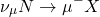Neutrinos, in particular neutrino-hadron collisions, are used as a proe of the inner structure of hadrons and QCD. Neutrino are neutral particles that only interact through the weak interaction and likely the gravitational force, so we can use them as microscopes to see the inner hadron structure.

5th. The mass difference between two kaon species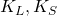,i.e.,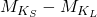is a test of CP violation in neutral kaon systems. In addition to it, it is also a test of-mixing.

Weak interactions and their CC and NC parts were discovered at CERN in 1973 (via the Gargamelle bubble-chamber collaboration). Since then, they have been tested successfully in a large set of experiments. Extra empirical evidence from the EW sector and its CC-NC interactions are provided by:

1) Reactions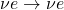,where N denotes a “nucleon” state and X an arbitrary set of particles with allowed quantum numbers.

2) Polarized electron-nuclei scatterin: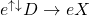3) Parity (and charge) violation experiments in atomic physics.

4) Electron-positron collisions at the Z-pole mass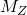, or energies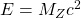, at LEP (before the LHC current era).

Remark: the self-interactions of the gauge bosons in the SM are PREDICTED by the underlying gauge invariance and they are tested experimentally. Experimental evidence for these self-interactions are, for example, processes like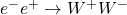This class of reactions were seen at LEP II and they are being observed at the LHC. There are three main diagrams for this collision. I mean Feynman diagrams. A technique that I should explain, but not today, as well. Two of them involve the 3-point interaction between a photon or Z boson and the W⁺W⁻ bosons.

After the Higgs-like particle discovery, one of the main tasks of physicists in the LHC is to determine their couplings to the SM fermions and to see IF the Higgs interaction vertices and the observed phenomenology match.

## Neutrino oscillations and masses

In 1998, the SuperKamiokande neutrino detector and later, independently, the SNO experiment determined for the first time that neutrinod do indeed flavor “oscillate” or “change” using solar neutrinos, i.e., SuperKamiokande and SNO observed the neutrinos emitted by the Sun, and they conclude they have mass. During the years 2001-2005, neutrino oscillations were studied for the first time at a “reactor experiment” called KamLAND, at Japan, measuring the neutrinos emitted by the different nuclear reactors from the country. They gave the best estimate of the difference in the squares of the neutrino masses. In May 2010, it was reported that physicists from CERN and the Italian National Institute for Nuclear Physics, in Gran Sasso National Laboratory, had observed for the first time a transformation in neutrinos in an accelerator experiment, providing further evidence that neutrino do have mass. Before 2012, the analogue matrix to the CKM matrix, the PMNS matrix, was determined up to one angle, the so-called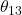. In 2012, this year, the Chinese experiment in Daya-Bay (a reactor experiment) and independently later by the south-korean reactor experiment RENO have provided evidence for a non-null and “large”. It has been confirmed by independent experiments. Here you are a nice summary with the last mixing angle they obtained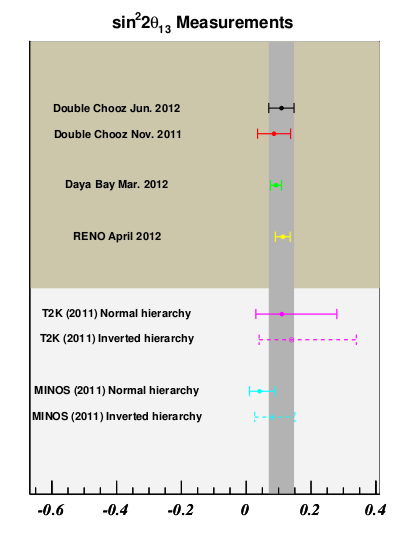We do know that neutrino have mass, a fact that is NOT the predictions of the SM. Indeed, the SM “predicts” massless neutrinos. Then, massive neutrinos are strong hints of new physics, whatever it is! However, their absolute scale is not known. We even ignore if neutrinos are even a weirder type of spinor fields called Majorana fields (essentially fermions that are their own antiparticles, unlike the other SM fermions). We have not observed (yet) CP violations in the leptonic sector, but the structure of the now “almost” complete PMNS matrix suggests that they have to exist. Furthermore, we do not know what type of spectrum neutrinos have (normal, inverted or quasidegenerated) or if there are heavy-right handed neutrinos (if they exist they are very massive and uncharged under the SM gauge group!) or sterile neutrinos ( neutrino species, not necessarily right-handed) uncharged under the SM gauge group. Neutrinos are the most mysterious particles of this Universe (if we neglect the graviton, the axion and other exotic particles whose probable existence has not been proved yet!).

The strongest upper limit on the masses of neutrinos comes from cosmological measurements:

1) The Big Bang model predits that there is a fiexe ratio between the number of neutrinos and the number of photons in the cosmic microwave background or CMB. If the total energy of neutrinos surpassed an average of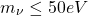per neutrino, or any number of this order of magnitude, there would be so much mass in the Universe that it would collapse. It is not collapsing. Even worst, supernovae IA measurements and complementary observations show that the Universe is expanding with an increasing velocity and positive acceleration! This is known as well from 1998…So, the Universe is not collapsing and the rate of the expansion is accelerating. Puzzling and mysterious stuff!

2) Cosmological data, such as the CMB radiation, galaxy clusters and the so-called Lyman-alpha forest indicate that the sum of the neutrino masses must be less that 1 eV ( the bounds are generally model dependent but there are stronger and softer bounds to this reference value of 1eV).

3) In 2009, data from gravitational lensing of a galaxy cluster also suggested a 1-2 eV upper mass bound for the neutrino species.

By the other hand, from the controlled measurements of solar neutrinos, reactor experiments and accelerator experiments ( specially those coming from solar, atmospheric and reactor/accelerator based experiments are crucial) provide some crucial mass differences for the neutrino species in the SM:

1) In 2005 KamLAND provided the estimate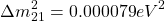2) MINOS experiment (accelerator based neutrino experiment, type long-baseline) providedThese data are being improved now, since the neutrino branch of the SM is a very active area of research and it uses very different methods in order to try to determine the neutrino mass absolute spectrum, its character and the spinorial nature of the neutrino field, yet uncovered (circa end of 2012). Such experiments include:

1) Nuclear beta decay (KATRIN, MARE,…).

2) Neutrinoless double beta decay (e.g., GERDA, CUORE/CUORICINO, NEMO-3, and many others).

3) Neutrino telescopes (IceCube, ANTARES,…)

Neutrinos are alive! 🙂 But let the Higgs bosons be with you!Stay tuned!

This site uses Akismet to reduce spam. Learn how your comment data is processed.